# Hyperons - Review up to the Pion Family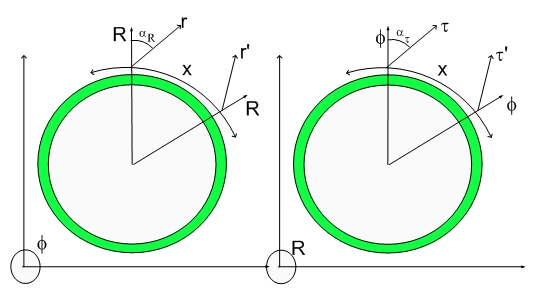Hyperon Review:

Let's take a look on the Fundamental Dilator and their relatives - The Hyperons.

The fundamental dilator is what we call a Proton or an Electron. Depending upon which phase is in phase with the Hyperspherical Lightspeed Expanding Universe one can have one or the other.

Transmutation transions can connect dimensional chords (each dimensional chord expresses the four phases of the fundamental dilator) and change the character of the dilator. I was able to create a full replacement for the Standard Model that makes use of dimensional chords and simple transmutation transitions/ transmutation chords.
Let take a look at the Fundamental Dilator once again.

The Electron(Proton):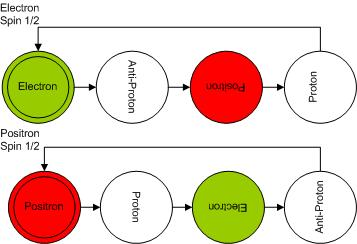The fundamental dilator is a 4D coherence between stationary states of deformation. The deformation of space is quantized, that is, can be represented as states in a 4D double potential well.

The respective coherences for Electron and Proton are shown below: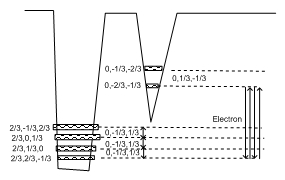and for a Proton: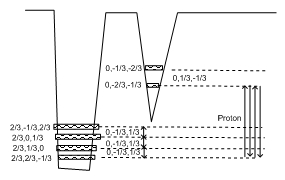Notice that the Proton and Electron coherences differ only by which is the initial (in phase) state in the coherence. The states associated with the Electron (0,-2/3,-1/3) and (0,-1/3,-2/3) differ not by "energy" since they have the same 3D volume (the Hpergeometrical Universe equivalent to Energy is the 3D projection of the 4D Volume) but by time. It takes time to rotate the dilator around (at the speed of light) and that introduces an extra phase delay on the Tunnelling Process with respect to the Spinning Process. Remember that all particles in the Universe are described in terms of combinations of this two fundamental dilators and that they have Spin Half, that is, they spin at half the speed of tunneling...:) A nice touch, I might say...:)

The Neutron is the first dimmer or two chord sequence. Its Balls Diagram is shown below: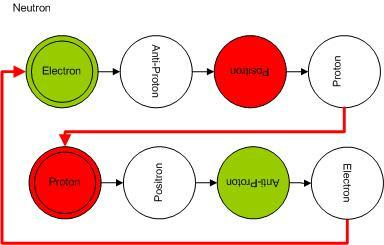Notice that I decided to use Red Balls for Positive and Green Balls for Negative moieties...:) Electrician Conventions... (never cut the Green Wire...)>:)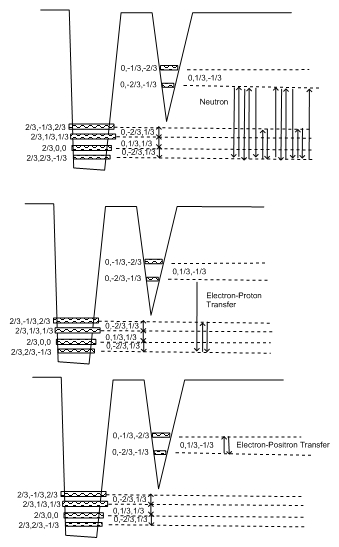Notice that the diagram above introduce two transmutation chords:

• Electron-Proton Transmutation Chord
• Electron-Positron Transmutation Chord

The Electron-Proton Transmutation Chord is present on the Neutron Model.

The dimmer character is derived from the impossibility to determine which dimensional note (Electron or Proton) is in phase with the Universe. Under these conditions the Neutron is represented by the two states (one starting with the electron and another starting with the proton) with half probability each one.

TheElectron-Positron Transmutation Chord was first shown in the Pion Family. I will replicate the Pion Family below for convenience.

Pion Plus and The Transmutation of Matter

Some time ago I mentioned that I would deliver
a Pedra filosofal or the Philosopher's Stone, the Alchemist dream of transmutation

Before you start making your plans for a rich retirement in the Bahamas, you might as well relax with some Pion Plus Music...:) There will be plenty of transmutation there...:)

Here are the decay equations associated with the Pion Brothers: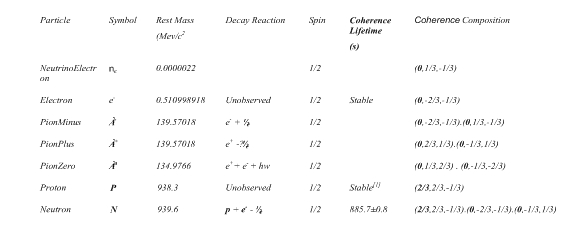Let's start with the Coherence Diagram containing the Dimensional Chords: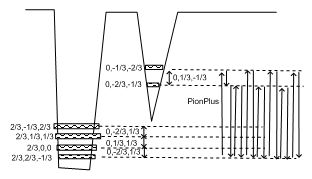One notices that the spectral content remains the same, that means that the decay products will differ only by phase. The front laden anti-proton to positron transmutation note generates the first positron of the sequence. Figure below shows this complex chord in a simpler manner: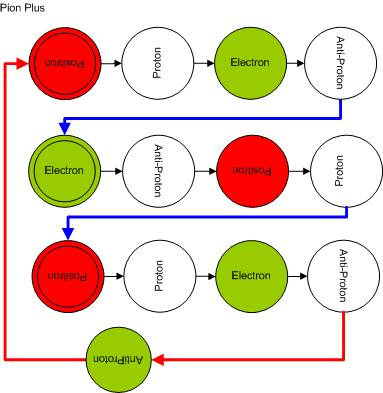If one compares it with the Pion Minus presented before, it is clear that they differ just by a phase. In the Pion Minus the initial phase is a electron while in the Pion Plus is is a positron. The phase difference is just 180 degrees tunnelling.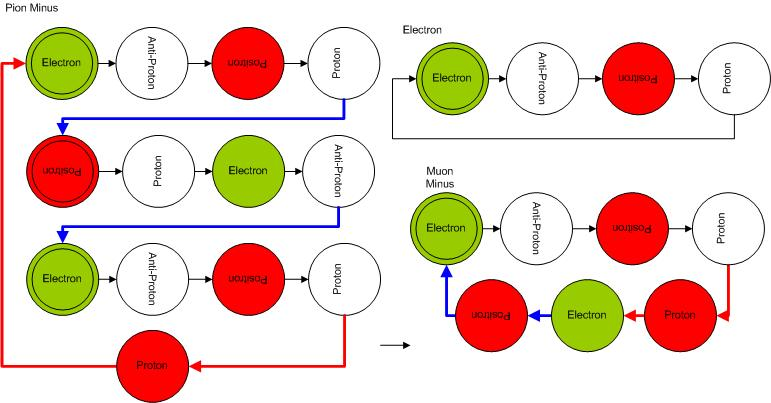Below is the Coherence Diagram for the Pion Zero: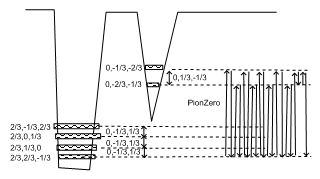and its corresponding balls diagram...:)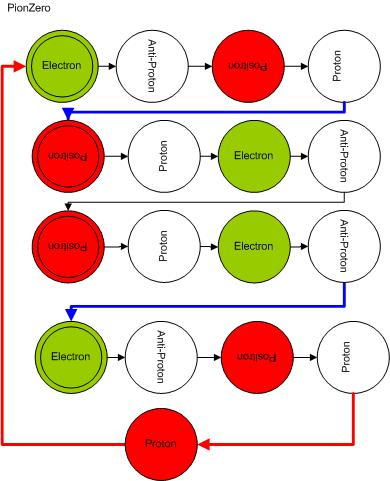You might notice that the Pion Zero looks like two justaposed electron-positron pairs of notes. You might ask how those two notes pairs don't anihiliate or collapse? There is a simple answer, they are not in the same time, that is, they are sequential notes. The other half of the answer has to do with angular momentum, for anti notes to continue existing or be something in life, they have to carry a total momentum different than zero. The idea is that if in the electron note one compresses 4D space and in the positron note one dilates the same space by the same amount, the net work is zero - work, energy and deformational /twisting energy are the same. This would mean that the note sequence could be eliminated or added without changing particle. Now if the zero note pair has angular momentum, then it cannot be eliminated willy-nilly.

Notice that the Blue and Red lines have spin quarter or spin -1/4 and their spin together with the half spins of the other fundamental dilator moieties add together to the total "particle" spin. Simple reasoning can provide the different spin configurations. Notice that the annihilation of transmutation spins also eliminates the dephasing associated with the involved transmutation transitions and thus affects the mass of the particle. This means that one could have two multichords that differ only by 3D mass while having the same 4D mass, total spin etc.

In the Silver Surfer and throughout the Blog, I explained where the nuclear energy is stored. The dephasing associated with transmutation transitions (Blue and Red lines) change the 3D shadow of the 4D dilator by changing the angle of the Fabric of Space. I already told you. The relationship between dephasing and the angle of the Fabric of Space should be patently clear to a four years old child by now....:)

There will be more on transmutation notes in the near future.

Cheers,

MP

Currently unrated

### New Comment

required

required (not published)

optional

required